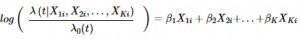# Hazard Ratio: Definition, Examples & Log of the Hazard

Design of Experiments > Hazard Ratio

## What is a Hazard Ratio?The hazard ratio is a comparison between the probability of events in a treatment group, compared to the probability of events in a control group. It’s used to see if patients receiving a treatment progress faster (or slower) than those not receiving treatment.

As a formula, the hazard ratio, which can be defined as the relative risk of an event happening at time t, is:
λ(t) / λ0.

• A hazard ratio of 3 means that three times the number of events are seen in the treatment group at any point in time. In other words, the treatment will cause the patient to progress three times as fast as patients in the control group. Any ratio above 1 generally means that the treatment group healed faster or had a slower time to an event.
• A hazard ratio of 1 means that both groups (treatment and control) are experiencing an equal number of events at any point in time.
• A hazard ratio of 0.333 tells you that the hazard rate in the treatment group is one third of that in the control group.

What the “event” is depends on the type of study. For example, it may be death, a cure, or another event–like a stroke.

Hazard ratios can be used to:

• Show the relative risk of a complication (like developing a side effect from a drug) in treatment group vs. control group.
• Show whether a treatment shortens an illness duration.
• Show which individuals are more likely to experience an event first.

While a hazard ratio is similar to a relative risk ratio, it isn’t exactly the same. Let’s say a clinical trial investigated survival rates for two drugs (A and B). The reported hazard ratios and relative risk ratios were both 3.

• The relative risk ratio tells you that the risk of death is three times higher with drug A than with drug B over the entire period of the study (i.e. it’s cumulative).
• The hazard ratio tells you that the risk of death is three times higher with drug A than with drug B at any particular point in time.

When evaluating hazard ratios, it’s recommended that you also use another measure such as median survival time, overall survival, or time to progression. These factors can provide insights about clear benefits (i.e. survival time is increased on average by 180 days).

## Hazard Log

Logarithmic transformation is often used in survival analysis to adjust a certain variable for covariates, decrease the effects of outliers and make the data more like a normal distribution (which makes it easier to analyze).

As a formula, the hazard function (i.e. the risk of a certain event at time t), can be expressed in terms of the log of the hazard (Abramson & Abramson, 2001):
Log of hazard = log(a) + b1x1…+ bkxk
The model is additive with respect to the hazard log, as demonstrated by the formula; this means that the log of the hazard ratio can be transformed to the hazard ratio using exponents. The model is also multiplicative, with respect to the hazard.

“Log of the hazard”, or “hazard log” could also be short for natural log of the hazard ratio itself (i.e. the hazard function divided by the baseline hazard function at time t):The above formula is a combination of parameters and regressors.

## References

Beyer, W. H. CRC Standard Mathematical Tables, 31st ed. Boca Raton, FL: CRC Press, pp. 536 and 571, 2002.
Agresti A. (1990) Categorical Data Analysis. John Wiley and Sons, New York.
Kotz, S.; et al., eds. (2006), Encyclopedia of Statistical Sciences, Wiley.
Vogt, W.P. (2005). Dictionary of Statistics & Methodology: A Nontechnical Guide for the Social Sciences. SAGE.

CITE THIS AS:
Stephanie Glen. "Hazard Ratio: Definition, Examples & Log of the Hazard" From StatisticsHowTo.com: Elementary Statistics for the rest of us! https://www.statisticshowto.com/hazard-ratio/